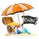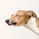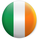3625 views
3625

Here is a fundamental version of a moving average cross trading strategy that works on 1H and 4H timeframes.

It's very simple and would be well paired with proper technical analysis and risk management.

I am looking to use this to alert me of potential setups. I could not get the alertcondition function to work properly. If anyone can help me with that please comment or message me.
```//@version=2
strategy(shorttitle = "Gamma pips EMA Cross", title="MA Cross", overlay=true)
s100sma = sma(close, 100)
s200sma = sma(close, 200)
s26ema = ema(close,26)
s12ema = ema(close,12)

plot(s100sma, color = green, linewidth = 5)
plot(s200sma, color = blue, linewidth = 5)
plot(s26ema, color = yellow, linewidth = 3)
plot(s12ema, color = red, linewidth = 3)
EMACross = plot(cross(s26ema, s12ema) ? s26ema : na, style = cross, linewidth = 5, color = red)
SMACross = plot(cross(s100sma, s200sma) ? s200sma : na, style = cross, linewidth = 5, color = white)

//============ signal Generator ==================================//
EMACrossover = crossover(s26ema, s12ema) //if yellow cross and is above red ->SELL
EMACrossunder = crossunder(s26ema, s12ema) //if yellow cross and is below red ->BUY
SMACrossover = crossover(s100sma, s200sma) //green crosses above blue ->Buy
SMACrossunder = crossunder (s100sma, s200sma) //green crosses below below ->Sell
price = close
BuyCondition = (EMACrossunder) and (price >= s100sma)
SellCondition = (EMACrossover) and (price <= s100sma)

///Short signal------//
if(SellCondition)
strategy.order("SELL ema crossover", strategy.short)

```If you have yet to figure this out, I would be happy to helpI'm sure you've figured this out after 5 months, but for anyone having the same problem I'm pretty sure it's because alertcondition doesn't work if the script is a strategy. Only if it's a study (the relevant part is mentioned on line 2) - I'm not fully confident this is the case, but there's so like Pine script info available online I figured I'd try and contribute.Hello,

Were you able to get a solution to this? I have a project I'd like to implement (pay for someone to develop) and it depends on being able to alert by sms. Just wondering if you solved it? - thanks! Also, how do I find programmers on here who are available for hire?